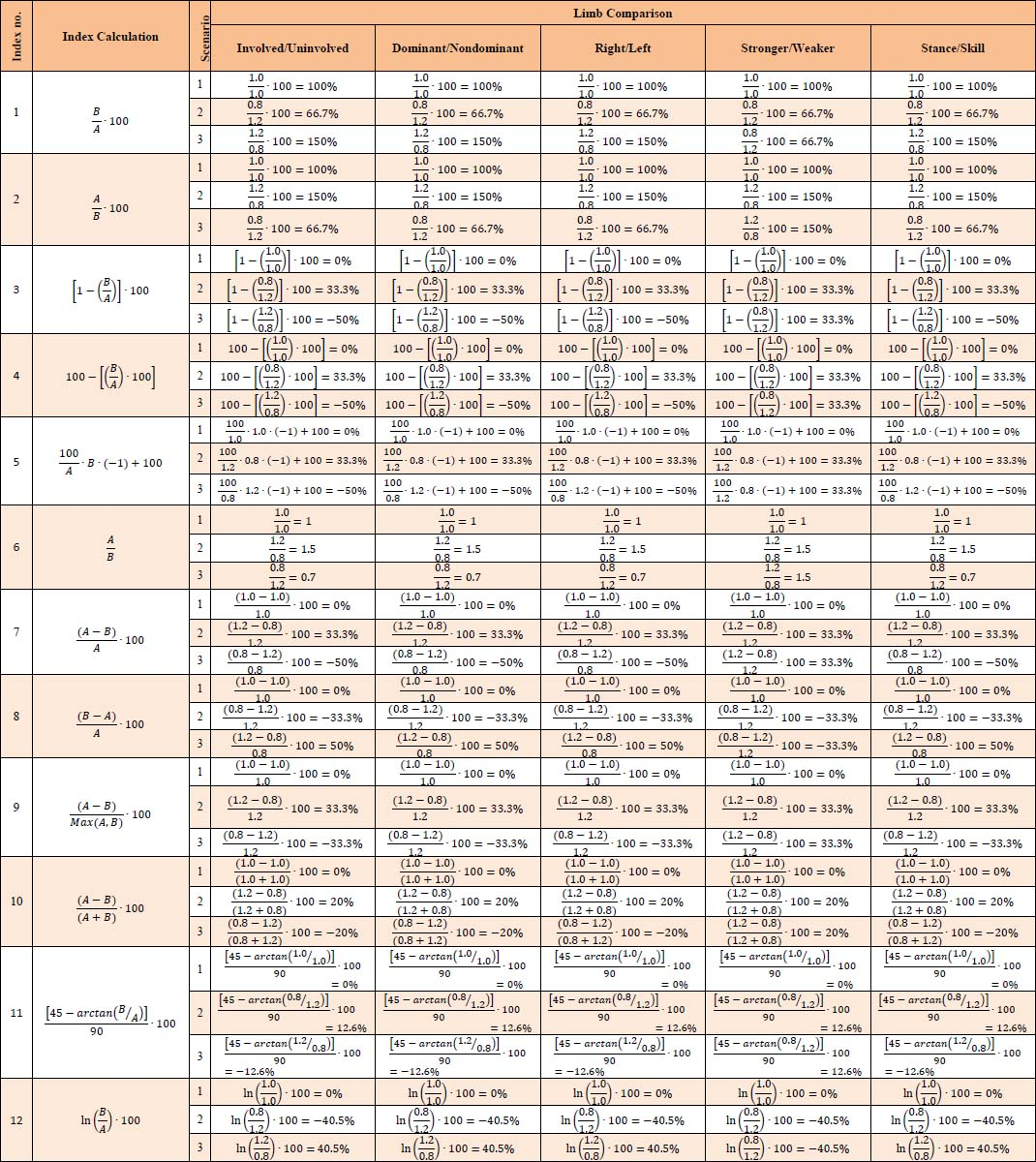Supplementary Material Table S1. Worked examples of hypothetical asymmetry scores for each index calculation across three scenarios: 1) limb symmetry, where A=B, 2) limb asymmetry, where A>B, or 3) limb asymmetry where A<B. Hypothetical peak torque values of 1.0Nm.kg-1, 1.2Nm.kg-1 and 0.8Nm.kg-1 were used.Limb A = uninvolved, dominant, right, stronger, or stance limb value, and Limb B = involved, nondominant, left, weaker, or skill limb value